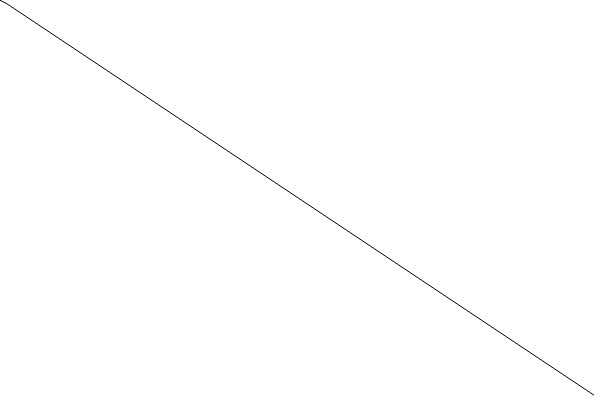# Define chart context

As we preivously mentioned, Plotters is drawing library. In theory, you should be able to draw any data plot based on the Plotters drawing API. On the top of drawing API, we provide the chart context, which creates a friendly environment for data visualization.

## Create a chart context from a drwaing area

`ChartBuilder` is the builder type to create a chart context.

The following code shows how to make a chart context that is using a 2D cartesian coordinate in which both X and Y axis is ranged 0 to 100.

``````use plotters::prelude::*;

fn main() {
let drawing_area = BitMapBackend::new("images/2.0.png", (1024, 768))
.into_drawing_area();

let _chart = ChartBuilder::on(&drawing_area)
.build_cartesian_2d(0..100, 0..100)
.unwrap();
}
``````

## Draw series on to the chart context

Once we have the chart context, we can put series on it. In this example, we use the `LineSeries` type to draw a line series on the chart.

``````use plotters::prelude::*;

fn main() {
let drawing_area = BitMapBackend::new("images/2.1.png", (600, 400))
.into_drawing_area();

drawing_area.fill(&WHITE).unwrap();

let mut chart = ChartBuilder::on(&drawing_area)
.build_cartesian_2d(0..100, 0..100)
.unwrap();

chart.draw_series(
LineSeries::new((0..100).map(|x| (x, 100 - x)), &BLACK),
).unwrap();
}
``````

This code will produce the following figure.